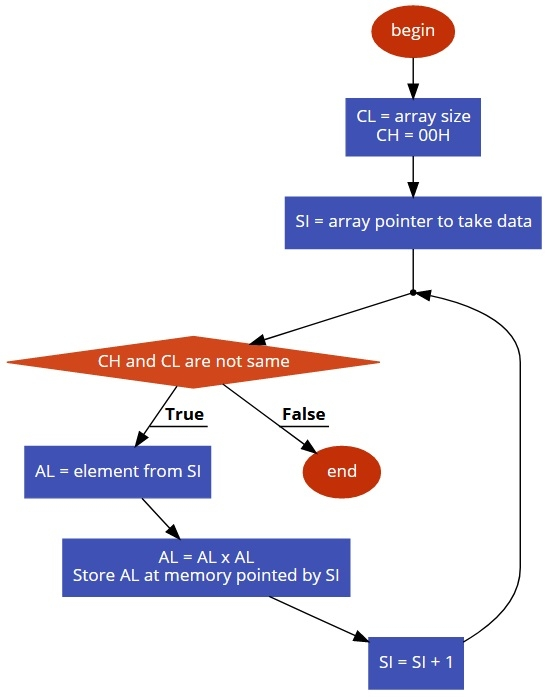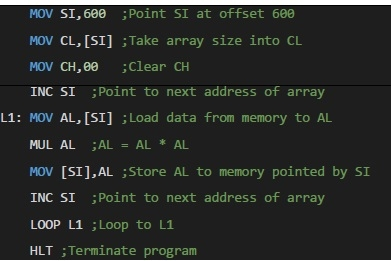# 8086 program to determine squares of numbers in an array of n numbers

MicroprocessorMicrocontroller8086

In this program we will see how to find the squares of n numbers stored in an array.

## Problem Statement

Write 8086 Assembly language program to calculate square of each numbers stored in an array of size n. The array size is stored at location offset 600, and Numbers are stored at 601 onwards.

## Discussion

To solve this problem, we are taking the size of the array into the CL register, and make CH = 00H for counting. Now from each location take the number into accumulator, to make square, we have to multiply it two times. so we are multiplying AL with AL. Thus the square is generated. After that it is again stored into memory location.

Data

600
05
601
02
602
03
603
05
604
01
605
04

## Flow Diagram## ProgramData

601
04
602
09
603
19
604
01
605
10